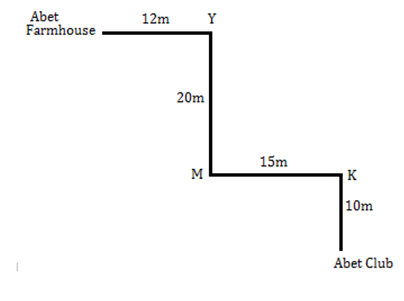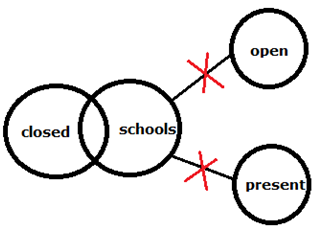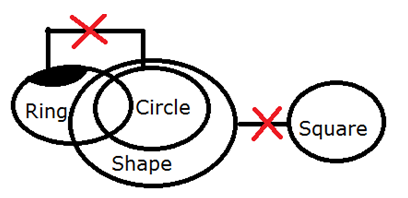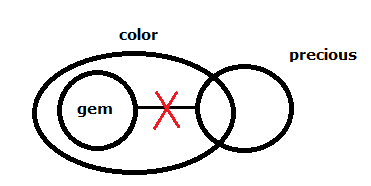# IBPS Clerk Prelims Reasoning (Day-10)

Dear Aspirants, Our IBPS Guide team is providing new series of Reasoning Questions for IBPS Clerk Prelims 2020 so the aspirants can practice it on a daily basis. These questions are framed by our skilled experts after understanding your needs thoroughly. Aspirants can practice these new series questions daily to familiarize with the exact exam pattern and make your preparation effective.

Start Quiz

Number series

Direction (1-4): Study the following information carefully and answer the questions given below.

281   393   928   572   743

1) If all the numbers are arranged in descending order from left to right, then which of the following will be the product of first and last digits of the number which is second from the right end?

A) 2

B) 9

C) 72

D) 21

E) 14

2) If the position of the first and third digits of each numbers is interchanged, what will be the sum of the first and second digits of the third lowest number thus formed?

A) 11

B) 10

C) 12

D) 7

E) 9

3) What will be the resultant if the first digit of the highest number is divided by the third digit of the second lowest number?

A) 4

B) 3

C) 2

D) 1

E) None of these

4) If all the digits in each of the numbers are arranged in descending order within the number from left to right, then which of the following number forms the second lowest?

A) 928

B) 281

C) 743

D) 572

E) 393

Direction sense

Direction (5-7): Study the following information carefully to answer the given question:

Abet walks 10m in the north from his club and reaches to point K. Now, he takes his left turn and goes 15m to reach point M. After that, he takes his right turn and goes 20m to reach point Y. From point Y, Abet takes his left turn and goes 12m to reach his farmhouse.

5) What is the total distance covered by Abet?

A) 35m

B) 45m

C) 57m

D) 47m

E) 67m

6) Point K is in which direction with respect to Point Y?

A) South-west

B) North

C) South

D) North-east

E) South-east

7) If Point D is 30m south from Abet farmhouse, then the shortest distance between Point D and Abet Club?

A) 22m

B) 25m

C) 27m

D) 15m

E) 20m

Syllogism

Direction (8-10): Read the conclusions and then decide which of the given conclusions logically follows from the given statements disregarding commonly known facts.

8) Statements:

No school is open

Some schools are closed

Some schools are not present

Conclusion:

I). Some present are closed

II). Some open can be closed

A) If Only conclusion I follows.

B) If Only conclusion II follows.

C) If either I or II follows.

D) If neither I nor II follows.

E) If both I and II follows.

9) Statements:

Only a few rings are circle

All circles are shape

No shape is square

Conclusion:

I). Some rings can be square

II). Some rings are not square

A) If Only conclusion I follows.

B) If Only conclusion II follows.

C) If either I or II follows.

D) If neither I nor II follows.

E) If both I and II follows.

10) Statements:

Some gem is not precious

All gems are color

Some color is precious

Conclusion:

I). All colors are gem

II). All color are precious

A) If Only conclusion I follows.

B) If Only conclusion II follows.

C) If either I or II follows.

D) If neither I nor II follows.

E) If both I and II follows.

Directions (1-4) :

928   743    572    393   281

Second from the right -> first*last digit -> 3*3=9

182   393    829   275   347

Third lowest number -> 347 -> first digit + second digit -> 3+4=7

9/3=3

281   393   928   572    743

821   933   982   752    743

Directions (5-7) :12+20+15+10=57

Directions (8-10):